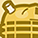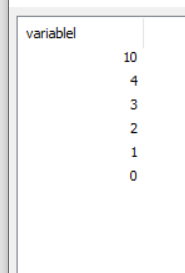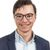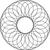# New to Qlik Sense

If you’re new to Qlik Sense, start with this Discussion Board and get up-to-speed quickly.

Announcements
LINKEDIN LIVE: Democratizing data to enhance customer-centricity. JULY 29TH REGISTER TODAY
cancel
Showing results for
Did you mean:Contributor III

## How to find 4th max value from list of variables in qlik sense ?

Dear All,

I have 6 numeric variables say vA,vB,vC,vD,vE,vF . I want to find 4 th max value from this list.

Example  Rangemax(2,1,6,8,9,3) gives 9 as max but I need 3 as 4th max value . Kindly suggest how to achieve same .

Labels (2)

• ### Qlik Sense

6 RepliesMaster II

several possibilities for example:

`````` let vA=1;
let vB=2;
let vC=4;
let vD=3;
let vE=0;
let vF=10;

Data:
load '\$(vA)' as variablel autogenerate 1;
load '\$(vB)' as variablel autogenerate 1;
load '\$(vC)' as variablel autogenerate 1;
load '\$(vD)' as variablel autogenerate 1;
load '\$(vE)' as variablel autogenerate 1;
load '\$(vF)' as variablel autogenerate 1;

output:
noconcatenate
load * resident Data order by variablel DESC;

drop table Data;

let V4th=peek('variablel',3,'output');``````v4th->2

Regards,
Taoufiq ZARRA

"Please LIKE posts and "Accept as Solution" if the provided solution is helpful "

(you can mark up to 3 "solutions") 😉Contributor III
Author

mostly I want to write in chart expressionPartner

You can make use of the following function:

https://help.qlik.com/en-US/sense/April2020/Subsystems/Hub/Content/Sense_Hub/ChartFunctions/BasicAgg...

you can specify which rank you want (nth largest number) which is in your case 4.

Hope this helps;)Creator II

Max(ValueList(\$(vA), \$(vB), \$(vC), \$(vD), \$(vE), \$(vF)), 4)Contributor III
Author

Thank you
It solved half of my problems.

For example one variable vA = 10 , vB = 30 , vC = count(EmpID) these three variables are created using  create new variable option .

Now If I will print it is showing \$(vC) = 45 and is in numeric format(checked by isNum() option )

but  Max(valueList( \$(vA) ,\$(vB) ,\$(vC) )) does not give any solution in chart expression while

Max(valueList( \$(vA) ,\$(vB) )) give  30 as max value in same chart expression. What I got to know using count() inside Max () might be the issue seems though.

Kindly suggest how to achieve same as \$(vC) = count(EmpID) and I need 2nd max of these three variables

Max(valueList( \$(vA) ,\$(vB) ,\$(vC) ),2) but no luck .

as count is present inside \$(vC) , because of same Max () might not work so how to over-ride such scenario as I need comparison .Creator II

Something like this...

Option 1:

vC Definition: Count([EmpID])

Chart Expression: Max(ValueList(\$(vA), \$(vB), \$(=\$(vC))), 2)

Option 2:

vC Definition: =Count([EmpID])

Chart Expression: Max(ValueList(\$(vA), \$(vB), \$(vC)), 2)Tags
Community Browser